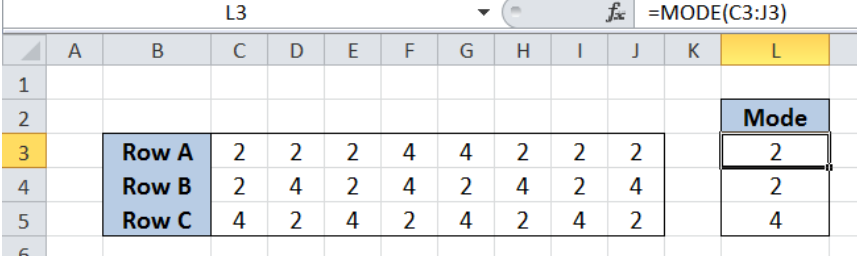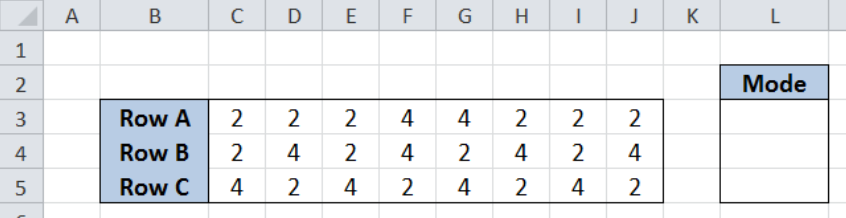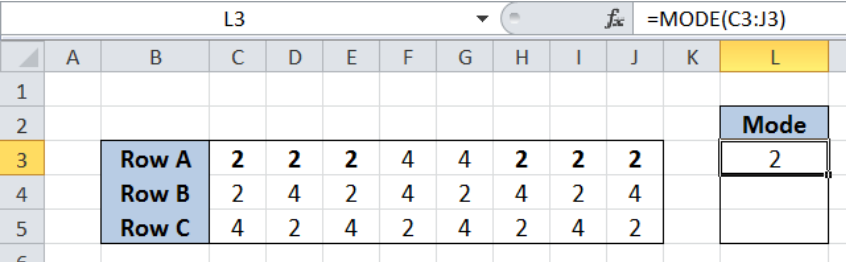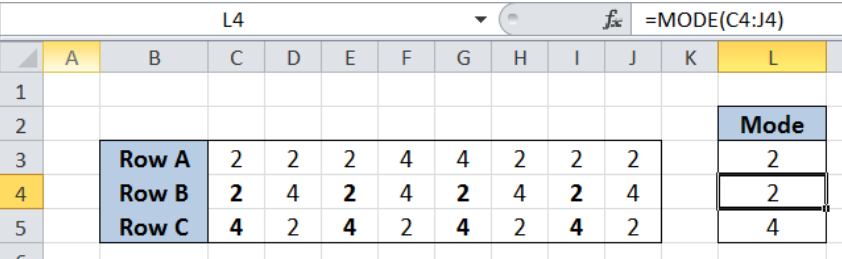Get instant live expert help with Excel or Google Sheets“My Excelchat expert helped me in less than 20 minutes, saving me what would have been 5 hours of work!”

#### Post your problem and you’ll get Expert help in seconds.

Your message must be at least 40 characters
Our professional Expert are available now. Your privacy is guaranteed.

# Most frequently occurring number

While working with Excel, we can easily determine the most frequently occuring number in a data set using the MODE function.  This step by step tutorial will assist all levels of Excel users in identifying the value that is repeated the most times in a range.Figure 1. Final result: Most frequently occuring number

## Syntax of the MODE function

MODE function returns the mode, or the number which appears most often in a set of numbers

Syntax: `=MODE(number1,[number2],...])`

The parameters are:

• number1 –  any value for which we want to determine the mode; could be an array or reference to cells containing numbers
• Only number1 is required; succeeding numbers are optional

## Setting up Our Data

Our table contains three rows, Rows A to C, containing a set of numbers from column C to column J.  We want to determine mode of each row in cells L3:L5.Figure 2. Sample data for most frequently occuring number

## Determine the mode in Row A

In order to determine the mode, or the number occurring most frequently in Row A, we follow these steps:

Step 1.  Select cell L3

Step 2.  Enter the formula: `=MODE(C3:J3)`

Step 3:  Press ENTERFigure 3. Entering the formula to determine the mode

Our array for the mode of Row A is the range C3:J3.  The final result in cell L3 is 2, which is the number repeated most often (6 times) in Row A.

## More than 1 mode in a data set

There can be more than one mode in a data set.  However, the MODE function is limited to show only one mode, which is the mode that first occurs in the data set.

Example:

Let us take note of Rows B and C.  Both rows have numbers 2 and 4, each number occurring four times in each row.  To determine the mode for Rows B and C, enter the following formulas:

In cell L4, enter the formula:  `=MODE(C4:J4) `

In cell L5, enter the formula:  `=MODE(C5:J5) `

As shown below, the mode of Row B is 2, because the number 2 occurs before the number 4 in Row B. On the other hand, the mode in Row C is 4.Figure 4. Output when there are two modes: MODE function returning the first mode

Most of the time, the problem you will need to solve will be more complex than a simple application of a formula or function. If you want to save hours of research and frustration, try our live Excelchat service! Our Excel Experts are available 24/7 to answer any Excel question you may have. We guarantee a connection within 30 seconds and a customized solution within 20 minutes.

### Did this post not answer your question? Get a solution from connecting with the expert.Another blog reader asked this question today on Excelchat:## Subscribe to Excelchat.coAnother blog reader asked this question today on Excelchat: